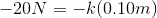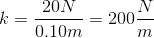# SAT II Physics : Spring Force

## Example Questions

### Example Question #1 : Forces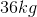girl bounces on a massless pogo stick. If the spring constant for the stick is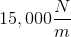, what is the maximum compression of the spring?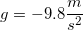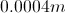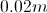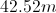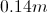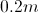Explanation:

There are two forces at work here: the force due to gravity and the restoring force of the spring. We can set these two forces equal to one another because the forces must be in equilibrium when the spring is compressed at its maximum point.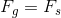Expand this equation by using the formulas for gravitational and spring force, respectively.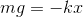Plug in our given values for the girl's mass, gravitational acceleration, and the spring constant. Using these values, we can solve for the displacement of the spring.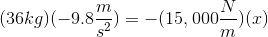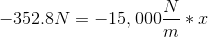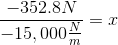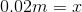### Example Question #11 : Mechanics

A certain spring has an equilibrium length of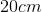. When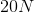of force are applied, the spring length becomes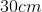. What is the spring constant?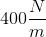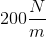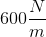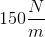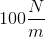Explanation:

The equation for spring force is: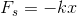The force applied to the spring will be equal and opposite the force of the spring itself. In this case, the force applied is, so the force of the spring will be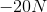.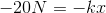The change in distance will be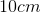, or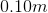. Using this value, we can solve for the spring constant.